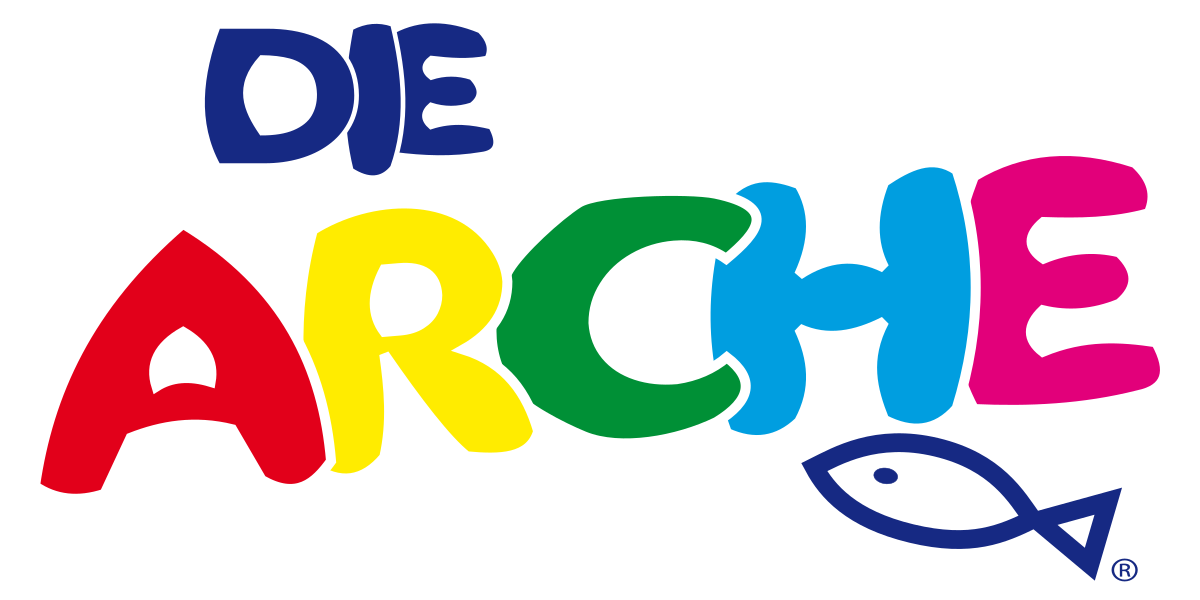# Conversion values ​​of measures and weight

### linear measure:

 1 inch (in.) = 2,54 cm 1 foot (ft.) = 12 inches = 30,48 cm 1 yard (yd.) = 3 feet = 91,44 cm

### weights:

 1 ounce (oz.av.) = 28,35 g 1 pound (lb. av.) = 16 ounces = 0,453 kg 2,2046 pounds = 1 kg

### room dimensions:

 1 cubic inch (cu.in.) = 16,387 ccm 1 cubic foot (cu.ft.) = 1728 cubic inches = 0,765 cbm 1 cubic yard (cu.yd.) = 27 cubic feet = 2,832 cbm 1 register ton (reg.tn.) = 100 cubic feet = 2,832 cbm 1,1960 square yard = 1 qm

### Calculation basis for the chargeable weight in airfreight

For airfreight shipment's  the airfreight costs are based on the actual weight or the volume weight of the shipment. Please find herewith the formula which is used to calculate the volume weight:

1 ton = 1.000, - kg corresponding to 6 cbm
1.000, - kg: 6 cbm means about 167, - kg = 1 cbm
Therefore: cbm x 167, - kg = volume weight

Or

Length x width x height x number of packages = total cubic centimeters
Total cubic centimeter / 6000 = volume weight

### Calculation basis for the chargeable weight in seafreight

For seafreight shipment's the actual weight is based on the weight in tons or the volume in cbm.

The minimum factor for calculating seafreight is either 1.000, - kg = 1 ton or 1 cbm

For shipment's by train the costs are based on the actual weight or the volume weight of the shipment. Please find herewith the formula which is used to calculate the volume weight:

1 ton = 1.000, - kg corresponding to 2 cbm
1.000, - kg: 2 cbm means 500, - kg = 1 cbm
Therefore: cbm x 500, - kg = volume weight

Or

Length x width x height x number of packages = total cubic centimeters
Total cubic centimeters / 2000 = volume weight

When loading by train at least 1,000, - kgs or 2 cbm are charged as minimum!

Please note that in air and seafreight, but also in rail transport, the higher value is always charged.THE ARK is supported by us regularly with special actions